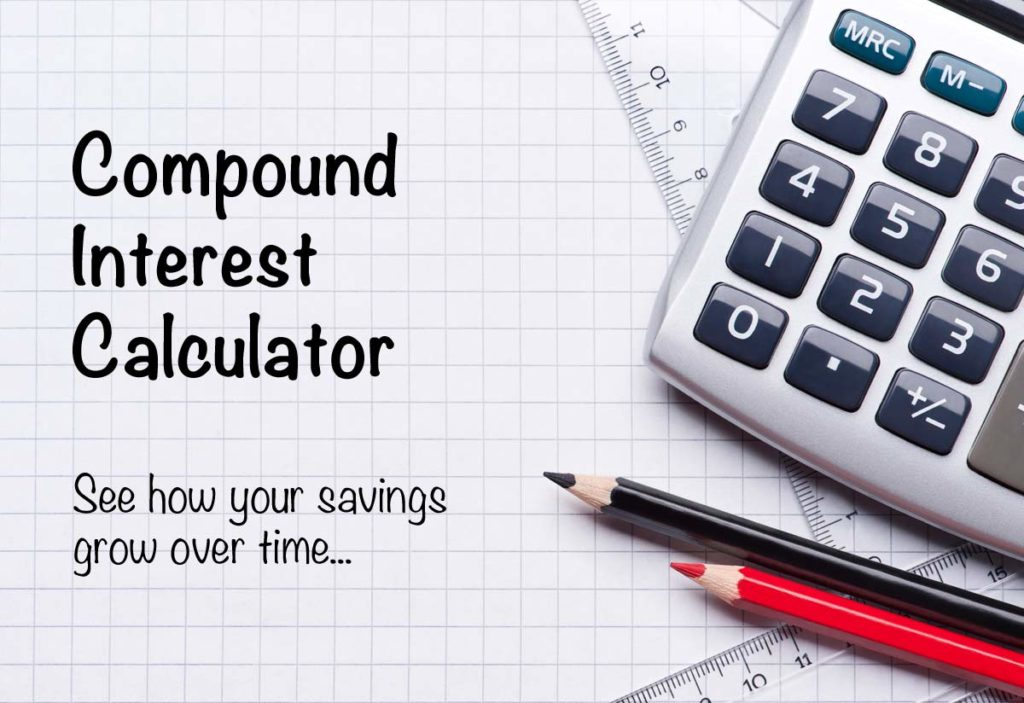# Compound Interest is either your best friend or your enemy. Here’s how to make it work for you597 Views

Compound interest is levied on interest and the principal amount. The interest from one term is added to the principal amount. In the next term, the interest is levied on the total amount. Interest is the charge a borrower must pay the lender for renting the money. Interest rate is the percentage at which interest is charge. There are two forms of interest, simple and compound interest.

In simple interest, the interest is charged only on the principal amount. However, in compound interest, the interest is charged on the total amount.

For example-

A person invests Rs. 100 at a compounded rate of 10% per annum for two years. After the first term, the profit is Rs. 10.

If it was charged at simple interest, then it will earn Rs. 10 after the next term. In simple interest, the profit will be Rs. 20 after 2 years. However, when charged at compound interest, the profit will be higher.

The return will be Rs. 10 after the first term. However, the principal amount will increase to Rs. 110 in the next term. After two years, the total amount will be Rs. 121. If the term is longer, then the yields are higher. Compound interest can earn very high profits for a person. It’s a situation when compound interest was a friend. However, it can also cause downfall.

Compound interest can be a person’s ally or enemy. It is a lender’s best friend. However, it is a borrower’s nightmare.

## Compound Interest as an Ally–

Compound interest is a good thing if you are the lender.When a person lends money on compound interest, they can earn higher profits as the interest is added on the principal amount after every term.

For example-

A person invests Rs. 10,000 in a fixed deposit for 4 years at an interest rate of 10% per annum.

• After the first term, the returns will be Rs. 1,000. The principal amount increases to Rs. 11,000.
• In the next term, the person will earn returns of Rs. 1,100. The principal amount is Rs. 12,100.
• In the next term, the returns will be Rs. 1,210. The principal amount increases to Rs. 13,310.
• After that term, the returns will be Rs. 1,331. The principal amount is Rs. 14,641.

After 4 years, compound interest will have earned the investor Rs. 14,641. However, the yields are higher when the term is longer. Compound interest gives higher returns when the investor puts a large amount. A person can save money to invest in instruments which offer compound interest.

## Compound Interest as an Enemy–

There are many benefits of investing with compound interest. However, things get bad when a person borrows on compound interest. If a person has borrowed a loan, then the interest amount can be more than the loan amount. It is due to compound interest.

For example-

A person buys a home appliance of Rs. 40,000 with a credit card. The person doesn’t have to pay the loan for three years and the interest rate is 6.08%.

• When the person starts paying the loan, the amount will not be Rs. 40,000, it will be Rs. 47,748 due to interest levied for three years.
• However, interest is charged every term until the borrower pays the loan.
• If the person pays the loan in 10 years, then they will pay more than Rs. 23,000 in interest.

If a person wants to earn higher yields, then it is important to have compound interest as a friend. However, borrowers can fend off against compound interest by paying the loan soon.

P = The Principal Amount

R = The Interest Rate

N = Term

T = Total Time

A = Total Amount

Compound Interest Calculator-

A = P(1 + R/N)NT

The most important factors that impact compound interest are the interest rate and term. The profits from compound interest will be higher if there are more terms. Also, the total amount will be high if the interest rate is high.

Compound interest has a big impact on investment as it can earn high profits. However, a person can fend off against its danger by paying off debts soon.